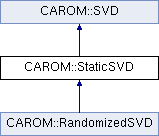libROM  v1.0 Data-driven physical simulation library
CAROM::StaticSVD Class Reference

`#include <StaticSVD.h>`

Inheritance diagram for CAROM::StaticSVD:## Public Member Functions

~StaticSVD ()

virtual bool takeSample (double *u_in, double time, bool add_without_increase=false)
Collect the new sample, u_in at the supplied time. More...

virtual const MatrixgetSpatialBasis ()
Returns the basis vectors for the current time interval as a Matrix. More...

virtual const MatrixgetTemporalBasis ()
Returns the temporal basis vectors for the current time interval as a Matrix. More...

virtual const VectorgetSingularValues ()
Returns the singular values for the current time interval. More...

virtual const MatrixgetSnapshotMatrix ()
Returns the snapshot matrix for the current time interval. More...Public Member Functions inherited from CAROM::SVD
SVD (Options options)
Constructor. More...

~SVD ()

int getDim () const
Returns the dimension of the system on this processor. More...

int getNumBasisTimeIntervals () const
Returns the number of time intervals on which different sets of basis vectors are defined. More...

double getBasisIntervalStartTime (int which_interval) const
Returns the start time for the requested time interval. More...

bool isNewTimeInterval () const
Returns true if the next sample will result in a new time interval. More...

void increaseTimeInterval ()
Increase the number of time intervals by one.

int getNumSamples () const
Get the number of samples taken.

## Protected Member Functions

StaticSVD (Options options)
Constructor. More...

virtual void computeSVD ()
Gathers samples from all other processors to form complete sample of system and computes the SVD.

bool thisIntervalBasisCurrent ()
Checks if the basis vectors for this time interval are up to date. More...

void get_global_info ()
Get the system's total row dimension and where my rows sit in the matrix.

void delete_samples ()
Delete the samples from ScaLAPACK.

void delete_factorizer ()
Delete the factorizer from ScaLAPACK.

Broadcast the sample to all processors.

## Protected Attributes

std::unique_ptr< SLPK_Matrix > d_samples
Current samples of the system.

std::unique_ptr< SVDManager > d_factorizer
Factorization manager object used to compute the SVD.

bool d_this_interval_basis_current
Flag to indicate if the basis vectors for the current time interval are up to date.

int d_rank
The rank of the process this object belongs to.

int d_num_procs
The number of processors being run on.

std::vector< int > d_istarts
The starting row (0-based) of the matrix that each process owns. Stored to avoid an MPI operation to get this operation every time we scatter a sample.

std::vector< int > d_dims
The number of rows that each process owns. Stored to avoid an MPI operation to get this operation every time we scatter a sample.

int d_total_dim
The total dimension of the system (row dimension)

int d_nprow
The number of processor rows in the grid.

int d_npcol
The number of processor columns in the grid.

int d_blocksize
The block size used internally for computing the SVD.

int d_max_basis_dimension
The max number of basis vectors to return.

double d_singular_value_tol
The tolerance for singular values below which to drop vectors.Protected Attributes inherited from CAROM::SVD
int d_dim
Dimension of the system.

int d_num_samples
Number of samples stored for the current time interval.

int d_num_rows_of_W
Number of rows in right singular matrix.

const int d_samples_per_time_interval
The maximum number of samples to be collected for a time interval.

const int d_max_time_intervals
The maximum number of time intervals.

Matrixd_basis
The globalized basis vectors for the current time interval. More...

Matrixd_basis_right
The globalized right basis vectors for the current time interval. More...

Matrixd_U
The matrix U which is large. More...

Matrixd_W
The matrix U which is large. More...

Vectord_S
The vector S which is small. More...

Matrixd_snapshots
The globalized snapshot vectors for the current time interval. More...

std::vector< double > d_time_interval_start_times
The simulation time at which each time interval starts.

bool d_debug_algorithm
Flag to indicate if results of algorithm should be printed for debugging purposes.

## Friends

class BasisGenerator

## Detailed Description

StaticSVD implements the interface of class SVD for the static SVD algorithm. This algorithm is not scalable and is intended primarily as a sanity check of the incremental SVD algorithm.

## Constructor & Destructor Documentation

 CAROM::StaticSVD::~StaticSVD ( )

Destructor.

 CAROM::StaticSVD::StaticSVD ( Options options )
protected

Constructor.

Parameters
 [in] options The struct containing the options for this SVD implementation.
Options

## Member Function Documentation

 virtual const Vector* CAROM::StaticSVD::getSingularValues ( )
virtual

Returns the singular values for the current time interval.

Postcondition
thisIntervalBasisCurrent()
Returns
The singular values for the current time interval.

Implements CAROM::SVD.

 virtual const Matrix* CAROM::StaticSVD::getSnapshotMatrix ( )
virtual

Returns the snapshot matrix for the current time interval.

Returns
The snapshot matrix for the current time interval.

Implements CAROM::SVD.

 virtual const Matrix* CAROM::StaticSVD::getSpatialBasis ( )
virtual

Returns the basis vectors for the current time interval as a Matrix.

Postcondition
thisIntervalBasisCurrent()
Returns
The basis vectors for the current time interval.

Implements CAROM::SVD.

 virtual const Matrix* CAROM::StaticSVD::getTemporalBasis ( )
virtual

Returns the temporal basis vectors for the current time interval as a Matrix.

Postcondition
thisIntervalBasisCurrent()
Returns
The temporal basis vectors for the current time interval.

Implements CAROM::SVD.

 virtual bool CAROM::StaticSVD::takeSample ( double * u_in, double time, bool add_without_increase = `false` )
virtual

Collect the new sample, u_in at the supplied time.

Precondition
u_in != 0
time >= 0.0
Parameters
 [in] u_in The new sample. [in] time The simulation time of the new sample. [in] add_without_increase If true, then addLinearlyDependent will be invoked
Returns
True if the sampling was successful.

Implements CAROM::SVD.

 bool CAROM::StaticSVD::thisIntervalBasisCurrent ( )
inlineprotected

Checks if the basis vectors for this time interval are up to date.

Returns
True if the basis vectors for this time interval are up to date.

The documentation for this class was generated from the following file: